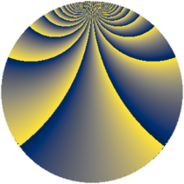# Properties

 Label 784.4.bhLevel $784$ Weight $4$ Character orbit 784.bh Rep. character $\chi_{784}(29,\cdot)$ Character field $\Q(\zeta_{28})$ Dimension $4008$ Sturm bound $448$

# Related objects

## Defining parameters

 Level: $$N$$ $$=$$ $$784 = 2^{4} \cdot 7^{2}$$ Weight: $$k$$ $$=$$ $$4$$ Character orbit: $$[\chi]$$ $$=$$ 784.bh (of order $$28$$ and degree $$12$$) Character conductor: $$\operatorname{cond}(\chi)$$ $$=$$ $$784$$ Character field: $$\Q(\zeta_{28})$$ Sturm bound: $$448$$

## Dimensions

The following table gives the dimensions of various subspaces of $$M_{4}(784, [\chi])$$.

Total New Old
Modular forms 4056 4056 0
Cusp forms 4008 4008 0
Eisenstein series 48 48 0

## Trace form

 $$4008q - 10q^{2} - 10q^{3} - 10q^{4} - 10q^{5} - 10q^{6} + 74q^{8} + O(q^{10})$$ $$4008q - 10q^{2} - 10q^{3} - 10q^{4} - 10q^{5} - 10q^{6} + 74q^{8} + 2q^{10} - 10q^{11} - 10q^{12} - 10q^{13} - 132q^{14} - 20q^{15} - 10q^{16} - 20q^{17} - 196q^{18} - 24q^{19} + 70q^{20} - 66q^{21} + 494q^{22} + 490q^{24} - 10q^{26} - 118q^{27} - 546q^{28} - 10q^{29} + 8q^{30} + 4416q^{31} - 990q^{32} - 20q^{33} - 698q^{34} - 490q^{35} - 266q^{36} - 10q^{37} + 210q^{38} + 830q^{40} + 666q^{42} - 10q^{43} + 1530q^{44} - 402q^{45} - 742q^{46} + 1860q^{47} - 1120q^{48} - 24q^{49} + 1372q^{50} + 1498q^{51} + 3194q^{52} - 10q^{53} + 1874q^{54} - 2928q^{56} + 3378q^{58} - 1386q^{59} - 4654q^{60} - 10q^{61} - 458q^{62} - 1396q^{63} - 1102q^{64} - 20q^{65} + 2786q^{66} - 1872q^{67} - 1788q^{68} - 118q^{69} + 330q^{70} - 2058q^{72} - 2166q^{74} + 382q^{75} - 7660q^{76} - 698q^{77} - 2366q^{78} - 48q^{79} - 13708q^{80} + 51496q^{81} - 8742q^{82} + 2430q^{83} + 11116q^{84} + 490q^{85} - 2068q^{86} + 760q^{88} - 6742q^{90} + 9254q^{91} + 3444q^{92} - 280q^{93} - 698q^{94} - 7748q^{95} + 12840q^{96} - 48q^{97} - 10688q^{98} - 2832q^{99} + O(q^{100})$$

## Decomposition of $$S_{4}^{\mathrm{new}}(784, [\chi])$$ into newform subspaces

The newforms in this space have not yet been added to the LMFDB.